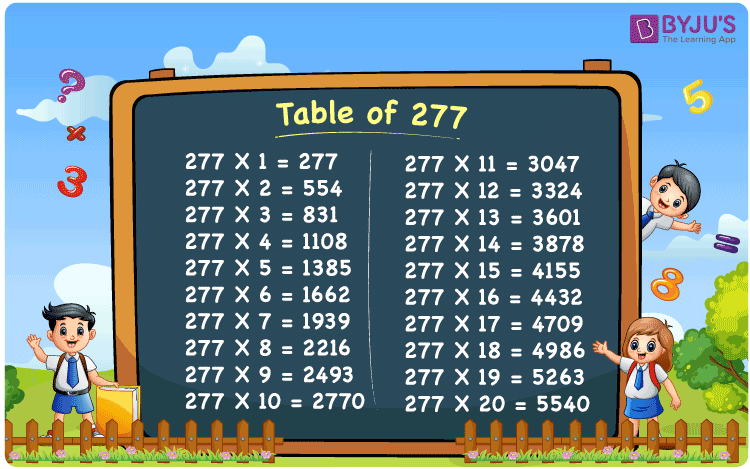Checkout JEE MAINS 2022 Question Paper Analysis : Checkout JEE MAINS 2022 Question Paper Analysis :

# Table of 277

The table of 277 is provided here for students to learn the 277 times table up to 20 times. Multiplication tables are essential to calculate the problems quickly. Students are highly recommended to follow the multiplication tables thoroughly to boost their performance in exams. Access the table of 277 at BYJU’S for quick reference while solving the problems.## What is the 277 Times Table?

The multiplication of 277 with any number is the 277 times table. The table of 277 can be obtained by multiplication and addition of 277 to a given number of times. Learn the table of 277 in both multiplication and addition form from the chart here.

 277×1 = 277 277 277×2 = 554 277 + 277 = 554 277×3 = 831 277 + 277 + 277 = 831 277×4 = 1108 277 + 277 + 277 + 277 = 1108 277×5 = 1385 277 + 277 + 277 + 277 + 277 = 1385 277×6 = 1662 277 + 277 + 277 + 277 + 277 + 277 = 1662 277×7 = 1939 277 + 277 + 277 + 277 + 277 + 277 + 277 = 1939 277×8 = 2216 277 + 277 + 277 + 277 + 277 + 277 + 277 + 277 = 2216 277×9 = 2493 277 + 277 + 277 + 277 + 277 + 277 + 277 + 277 + 277 = 2493 277×10 = 2770 277 + 277 + 277 + 277 + 277 + 277 + 277 + 277 + 277 + 277 = 2770

## Multiplication Table of 277

Learn the multiplication table of 277 from 1 to 20 times from the chart mentioned below.

 277 × 1 = 277 277 × 2 = 554 277 × 3 = 831 277 × 4 = 1108 277 × 5 = 1385 277 × 6 = 1662 277 × 7 = 1939 277 × 8 = 2216 277 × 9 = 2493 277 × 10 = 2770 277 × 11 = 3047 277 × 12 = 3324 277 × 13 = 3601 277 × 14 = 3878 277 × 15 = 4155 277 × 16 = 4432 277 × 17 = 4709 277 × 18 = 4986 277 × 19 = 5263 277 × 20 = 5540

## Solved Example on the Table of 277

Example 1:

What is 5 less than the 13th multiple of 277?

Solution:

13th multiple of 277 = 3601

5 less than the 13th multiple of 277 = 3601 – 5 = 3596

Hence, 5 less than the 13th multiple of 277 is 3596.

## Frequently Asked Questions on Table of 277

### Is 277 a prime number?

Since 277 has only two factors i.e. 1 and 277. It is a prime number.

### What number should we multiply by 277 to get 4155?

From the table of 277,
277 times 15 is 4155.
Hence, 277 has to be multiplied by 15 to get 4155.

### Calculate 277 times 14 minus 20 plus 200.

277 times 14 = 277 x 14 = 3878.
277 times 14 minus 20 plus 200 = 3878 – 20 + 200 = 4058.